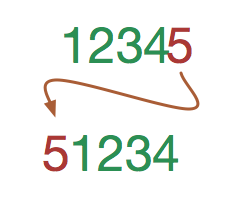# Rotate the integer to the left...When we rotate an integer, we take the last digit (right most) and move it to the front of the number. For example, if we rotate $12345$, we will get $51234$.

What is the smallest (positive) integer $N$, such that when $N$ is rotated, we obtain $\frac{2}{3} N$?

×

Problem Loading...

Note Loading...

Set Loading...